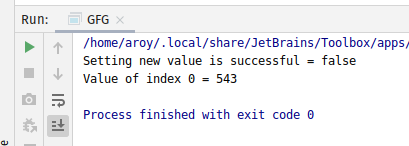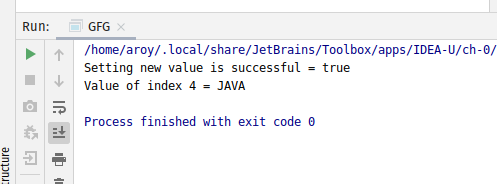Open in App
Not now

# AtomicReferenceArray weakCompareAndSetAcquire() method in Java with Examples

• Last Updated : 03 Jan, 2020

The weakCompareAndSetAcquire() method of a AtomicReferenceArray class is used to atomically sets the value of the element at index i to newValue to newValue for AtomicReferenceArray if the current value is equal to expectedValue passed as parameter. This method updates the value at index i with memory ordering effects compatible with memory_order_acquire ordering. This method returns true if set a new value to AtomicRefrence is successful.

Syntax:

```public final boolean
weakCompareAndSetAcquire(
int i, E expectedValue, E newValue)
```

Parameters: This method accepts:

• i which is an index of AtomicReferenceArray to perform the operation,
• expectedValue which is the expected value and
• newValue which is the new value to set.

Return value: This method returns true if set a new value to AtomicRefrence is successful.

Below programs illustrate the weakCompareAndSetAcquire() method:
Program 1:

 `// Java program to demonstrate ` `// weakCompareAndSetAcquire() method ` ` `  `import` `java.util.concurrent.atomic.*; ` ` `  `public` `class` `GFG { ` ` `  `    ``public` `static` `void` `main(String[] args) ` `    ``{ ` ` `  `        ``// create an atomic reference object. ` `        ``AtomicReferenceArray ref ` `            ``= ``new` `AtomicReferenceArray(``5``); ` ` `  `        ``// set some value ` `        ``ref.set(``0``, ``543``); ` `        ``ref.set(``1``, ``123``); ` `        ``ref.set(``2``, ``5443``); ` ` `  `        ``// apply weakCompareAndSetAcquire() ` `        ``boolean` `result ` `            ``= ref.weakCompareAndSetAcquire( ` `                ``0``, ` `                ``5443``, ` `                ``234``); ` ` `  `        ``// print value ` `        ``System.out.println( ` `            ``"Setting new value"` `            ``+ ``" is successful = "` `            ``+ result); ` ` `  `        ``System.out.println(``"Value of index 0 = "` `                           ``+ ref.get(``0``)); ` `    ``} ` `} `

Output:Program 2:

 `// Java program to demonstrate ` `// weakCompareAndSetAcquire() method ` ` `  `public` `class` `GFG { ` ` `  `    ``public` `static` `void` `main(String[] args) ` `    ``{ ` ` `  `        ``// create an atomic reference object.c ` `        ``AtomicReferenceArray ref ` `            ``= ``new` `AtomicReferenceArray(``5``); ` ` `  `        ``// set some value ` `        ``ref.set(``0``, ``"C"``); ` `        ``ref.set(``1``, ``"PYTHON"``); ` `        ``ref.set(``2``, ``"TS"``); ` `        ``ref.set(``3``, ``"C++"``); ` `        ``ref.set(``4``, ``"C"``); ` ` `  `        ``// apply weakCompareAndSetAcquire() ` `        ``boolean` `result ` `            ``= ref.weakCompareAndSetAcquire( ` `                ``4``, ` `                ``"C"``, ` `                ``"JAVA"``); ` ` `  `        ``// print value ` `        ``System.out.println( ` `            ``"Setting new value"` `            ``+ ``" is successful = "` `            ``+ result); ` ` `  `        ``System.out.println( ` `            ``"Value of index 4 = "` `            ``+ ref.get(``4``)); ` `    ``} ` `} `

Output:My Personal Notes arrow_drop_up
Related Articles